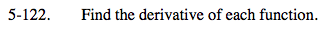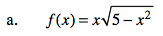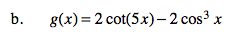### Home > CALC > Chapter 5 > Lesson 5.3.2 > Problem5-122

5-122.
1. Find the derivative of each function. Homework Help ✎

1.2. g(x) = 2 cot(5x) − 2 cos3 xThis derivative involves both the Product Rule and the Chain Rule.

$f'(x)=\frac{5-2x^{2}}{\sqrt{5-x^{2}}} \ \ \ \text{Show the steps!}$Be very careful to keep track of positive and negative coefficients.